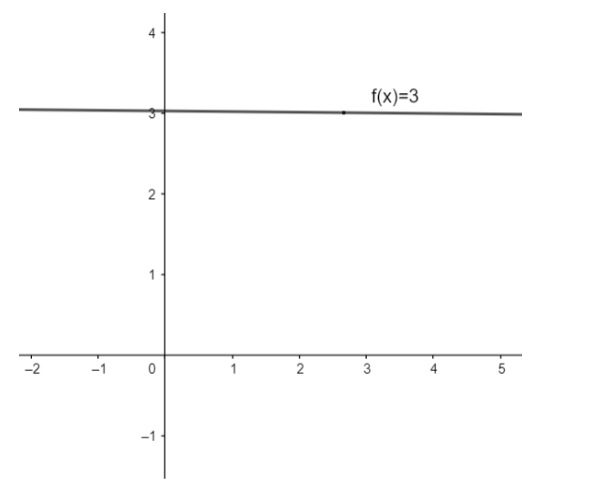Courses
Courses for Kids
Free study material
Offline Centres
MoreLast updated date: 28th Nov 2023
Total views: 382.2k
Views today: 8.82k

# Consider the constant function $f(x)=3$. Let us try to find its limit as $x=2$.Verified
382.2k+ views
Hint: The limit of a function exists only if left hand limit and right hand limit exist and both are equal.

Also, the value of the limit will be equal to the value of the right hand limit and (or) the left hand limit.
We know, the limit of a function exists only if the left hand limit and right hand limit exist and both are equal.
So, first, we will find the left hand limit of the function $f(x)=3$ at $x=2$.
We know, the left hand limit of a function $f(x)$ at $x=a$ is given as
$L.H.L=\underset{h\to 0}{\mathop{\lim }}\,f\left( a-h \right)$
So, left hand limit of the function $f(x)=3$ at $x=2$ is given as
$L.H.L=\underset{h\to 0}{\mathop{\lim }}\,f\left( 2-h \right)=3$[ $\because$$f(x)$ is a constant function, value of a function is 3 for all $x\in R$]
Now , we will find the right hand limit of the function $f(x)=3$ at $x=2$.
We know the right hand limit of a function $f(x)$ at $x=a$ is given as
$L.H.L=\underset{h\to 0}{\mathop{\lim }}\,f\left( a+h \right)$
So , right hand limit of the function $f(x)=3$ at $x=2$ is given as
$L.H.L=\underset{h\to 0}{\mathop{\lim }}\,f\left( 2+h \right)=3$
We can clearly see that both the left hand limit and the right hand limit of the function $f(x)=3$ exist at $x=2$.
Also, the left hand limit and the right hand limit of the function $f(x)=3$ at $x=2$ are equal.
Since, the value of $L.H.L=R.H.L$at $x=2$, hence, limit of the function $f(x)=3$ exists at $x=2$ and the value of limit of the function $f(x)=3$ at $x=2$ is $3$.

Note: The graph of the given function $f(x)=3$ is a straight line parallel to x-axis as shown in the figure.From the graph, we can clearly see that whether we approach $x=2$ from the left or right side, the value of the function is equal to $3$.
So, the value of left hand limit of the function $f(x)=3$ at $x=2$ is equal to $3$ and the value of right hand limit of the function $f(x)=3$ at $x=2$ is equal to $3$.
Hence, the value of $\underset{x\to 2}{\mathop{\lim }}\,f\left( x \right)$ is equal to $3$.